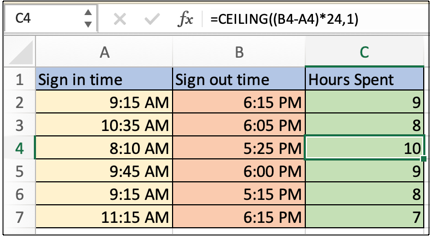# How to round time values up to nearest hour in ExcelIn this article, we will learn How to round time values up to the nearest hour in Excel.
Scenario:

Generally, we are stuck at time fields and not able to understand how to round up or round down time values. For example getting the count of hours spent by an employee in office. These tasks are very common to occur and we will use the CEILING function to round time values here. Let's learn How to use the function and an example to understand it briefly.

ROUND to nearest hour formula in Excel

The formula used here takes two time values signed out and sign in time entries. Formulate the two values using mathematical operators and round the result up to the nearest hour as explained below.

CEILING formula

 =CEILING ( ( out- in ) * 24 , 1 )

out : sign out time

* : operator to multiply

Example :

All of these might be confusing to understand. Let's understand how to use the function using an example. Here we have some employee time data and we need to calculate the hours spent by each employee in office as per data.

Use the formula:

 =CEILING((B4-A4)*24,1)As you can see all employee's timesheets are ready. If given the condition to filter we can use conditional formatting to highlight the defaulters or to find employees of month.

Here are all the observational notes using the formula in Excel
Notes :

1. The formula only works with numbers.
2. Careful while Input time values 04:10:22 means 4 hours, 10 minutes and 22 seconds.
3. You can use any of the ROUND functions like ROUNDUP, MROUND, CEILING or any other excel function, you are compatible with.

Hope this article about How to round time values up to the nearest hour in Excel is explanatory. Find more articles on calculating values and related Excel formulas here. If you liked our blogs, share it with your friends on Facebook. And also you can follow us on Twitter and Facebook. We would love to hear from you, do let us know how we can improve, complement or innovate our work and make it better for you. Write to us at info@exceltip.com.

Related Articles :

Converts decimal Seconds into time format : As we know that time in excel is treated as numbers. Hours, Minutes, and Seconds are treated as decimal numbers. So when we have seconds as numbers, how do we convert into time format? This article got it covered.

Calculate Minutes Between Dates & Time in Excel : calculating the time difference is quite easy. Just need to subtract the start time from the end time. Learn more about this formula clicking the link

minutes to hours and minutes : returns a numerical value which represents the same value but in decimal format in place of time format in excel.

Calculate hours between time in Excel : Calculate the hours between two time values in excel. find the time elapsed between end_time & start_time

Time difference in hours as decimal value : Time difference in hours using Excel MOD function and then we need to represent it in the decimal format.

SUM time over 9 hours in Excel : get the sum of time values which are above 9 hours

How to add Decimal Hours to Time : add numeric or decimal time to a time formatted value.

Popular Articles :

50 Excel Shortcuts to Increase Your Productivity : Get faster at your tasks in Excel. These shortcuts will help you increase your work efficiency in Excel.

How to use the VLOOKUP Function in Excel : This is one of the most used and popular functions of excel that is used to lookup value from different ranges and sheets.

How to use the IF Function in Excel : The IF statement in Excel checks the condition and returns a specific value if the condition is TRUE or returns another specific value if FALSE.

How to use the SUMIF Function in Excel : This is another dashboard essential function. This helps you sum up values on specific conditions.

How to use the COUNTIF Function in Excel : Count values with conditions using this amazing function. You don't need to filter your data to count specific values. Countif function is essential to prepare your dashboard.

Terms and Conditions of use

The applications/code on this site are distributed as is and without warranties or liability. In no event shall the owner of the copyrights, or the authors of the applications/code be liable for any loss of profit, any problems or any damage resulting from the use or evaluation of the applications/code.